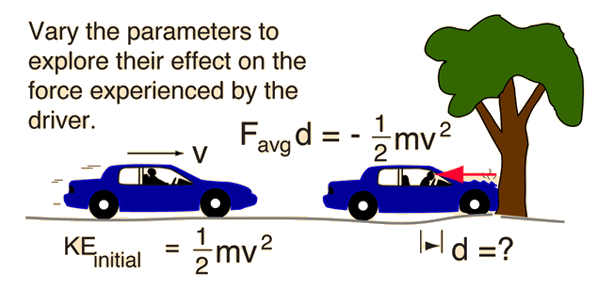# Force on Driver in Example Car Crash

For the car crash scenario where a car stops in 1 foot from a speed of 30 mi/hr, what is the force on the driver? Assume a 160 lb (mass = 5 slugs) driver.

If firmly held in non-stretching seatbelt harness: Stopping distance 1 ft.

 Deceleration = 967 ft/s2 = 294 m/s2 = 30 g's Force = 4813 lb = 21412 N = 2.4 tons Non-stretching seatbelt
If not wearing seatbelt, stopping distance determined by nature of collision with windshield, steering column, etc. : stopping distance 0.2 ft.
 Deceleration = 4836 ft/s2 = 1474 m/s2 = 150 g's Force = 24068 lb = 107059 N = 12 tons!! No seatbelt!
If seat belt harness stretches, increasing stopping distance by 50%: 1.5 ft.
 Deceleration = 645ft/s2 = 197 m/s2 = 20 g's Force = 3209 lb = 14274 N = 1.6 tons Stretching seatbelt
These calculated numbers assume constant deceleration, and are therefore an estimate of the average force of impact.Calculation of force on driver Non-stretching seatbelt Stretching seatbelt No seatbelt! Seatbelt and airbag
Index

Work-energy principle

 HyperPhysics***** Mechanics R Nave
Go Back

# Example of Calculation of Force on DriverIf the weight of the driver is N = lb, corresponding to
mass = kg = slugs,
and the car is traveling with a speed:
v = m/s = km/hr = mi/hr,
then a crash which stopped the driver in a distance d=m = ft
would result in an average impact force of

### Impact force F = N = lb = tons!!

You may change any data value. Clicking outside the box will then initiate a calculation of the impact force and conversion of the data value to the other types of units.

### How does a seatbelt change the impact force on the driver?

Index

Work-energy principle

Car crash scenario

 HyperPhysics***** Mechanics R Nave
Go Back